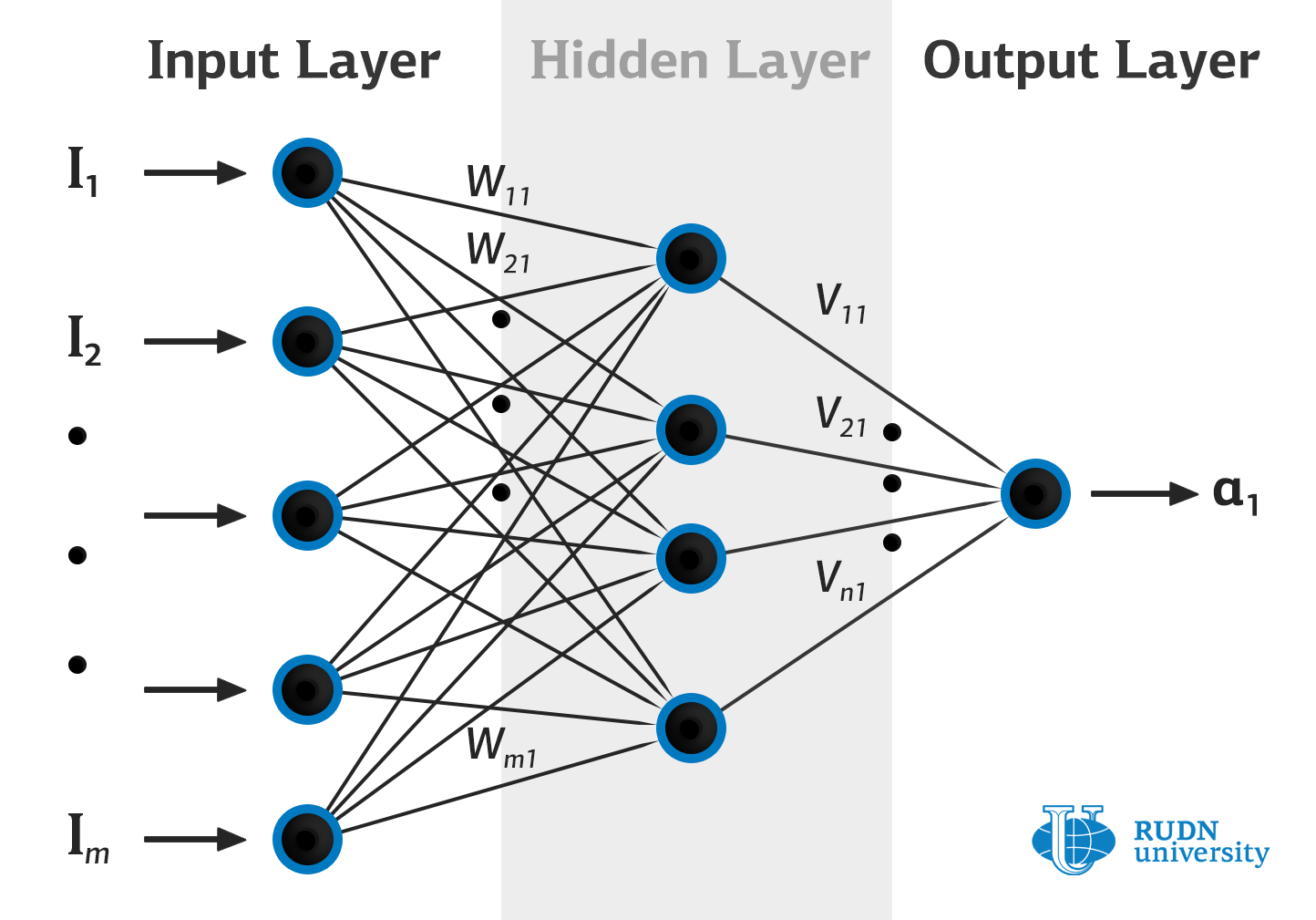Mathematicians from RUDN University and the Free University of Berlin proposed a new way of using neural networks for working with noisy high-dimensional data

# Mathematicians from RUDN University and the Free University of Berlin proposed a new way of using neural networks for working with noisy high-dimensional data

Mathematicians from RUDN University and the Free University of Berlin have proposed a new approach to studying the probability distributions of observed data using artificial neural networks. The new approach works better with so-called outliers, i.e. input data objects that deviate significantly from the overall sample.

The restoration of the probability distribution of observed data by artificial neural networks is the most important part of machine learning. The probability distribution not only allows us to predict the behaviour of the system under study, but also to quantify the uncertainty with which forecasts are made. The main difficulty is that, as a rule, only the data are observed, but their exact probability distributions are not available. To solve this problem, Bayesian and other similar approximate methods are used. But their use increases the complexity of a neural network and therefore makes its training more complicated.

RUDN University and the Free University of Berlin mathematicians used deterministic weights in neural networks, which would help overcome the limitations of Bayesian methods. They developed a formula that allows one to correctly estimate the variance of the distribution of observed data. The proposed model was tested on different data: synthetic and real; on data containing outliers and on data from which the outliers were removed. The new method allows restoration of probability distributions with accuracy previously unachievable.

The mathematicians of RUDN University and the Free University of Berlin used deterministic weights for neural networks and used the networks outputs to encode the distribution of latent variables for the desired marginal distribution. An analysis of the training dynamics of such networks allowed them to obtain a formula that correctly estimates the variance of observed data, despite the presence of outliers in the data. The proposed model was tested on different data: synthetic and real. The new method allows restoring probability distributions with higher accuracy compared with other modern methods. Accuracy was assessed using the AUC method (area under the curve is the area under the graph that allows making assessment of the mean square error of the predictions depending on the sample size estimated by the network as “reliable”; the higher the AUC score, the better the predictions).

The article was published in the journal Artificial Intelligence.News
All news
Science
15 Aug
Manager, secretary and welder are the most harmful professions for psychological health, a study by a RUDN University physician showed

RUDN University physician together with colleagues from Kazakhstan conducted the first study in Kazakhstan onthe standard of living on parameters related to physical and psychological health. Among other things, thestudy showed the three most harmful professions for psychological health.

Science
12 Aug
RUDN University scientist compares algorithms for solving the optimal control problem

The RUDN University scientist compared the performance of several algorithms for solving optimal control problems that arise everywhere, from economics to cosmonautics.

Science
22 Jul
DFDE-2022, conference of leading mathematicians ended at RUDN University

June 28 - July 5, RUDN University hosted the Ninth International Conference on Differential and Functional Differential Equations (DFDE-2022), a satellite of the International Congress of Mathematicians 2022, which takes place on July 6-14.